## LetsPlayMaths.Com

WELCOME TO THE WORLD OF MATHEMATICS

# Class 6 Decimal Numbers

Decimal Numbers

Decimal Numbers on Number Line

Decimal Number Place Value Table

Conversion of Decimal fractions into Decimal Numbers

Conversion of Decimal Numbers into Decimal fractions

Subtraction of Decimal Numbers

Multiplication of Decimal Numbers

Division of Decimal Numbers by Natural Number

Division of Decimal Numbers by Decimal Number

Decimal Numbers Test

Decimal Numbers Worksheet

## Decimal Numbers

A decimal number has two parts. One part to the left of decimal point and other to the right of decimal point. The part to the left of decimal point is known as whole number and the part to the right of decimal point is known as decimal part.

For example, in the 35.7, the whole number part is 35 and the decimal part is 7.

## Decimal Numbers on Number Line

Let us learn the representation of decimal numbers on a number line. Divide the unit length between 0 to 1 into 10 equal parts as shown below.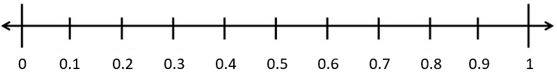Let us represent 0.5 on a number line. We know that 0.5 is more than 0 but less than 1. If we move 5 parts towards right from 0, then we can point 0.5 on the number line as shown in the below figure.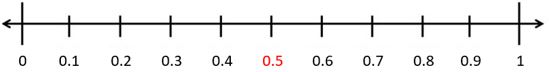Let us represent 1.8 on a number line. We know 1.8 is more than 1 but less than 2. If we move 8 parts towards right from 1, then we can point 1.8 on the number line as shown below.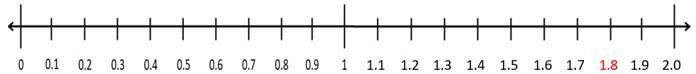## Decimal Number Place Value Table

Values ... Thousands Hundreds Tens Ones Tenths(110) Hundredths(1100) Thousandths(11000) ...
25.4 2 5 4
256.35 2 5 6 3 5
5782.056 5 7 8 2 0 5 6
25.004 2 5 0 0 4

## Conversion of Decimal fractions into Decimal Numbers

Count the number of zeros in the denominator and then count the same number of digits in the numerator starting from the unit digit and moving to the left, and then place the decimal point. Let's see some examples.

Example 1. Convert 2510 into decimal number.
Solution. 2510 = 2.5

Example 2. Convert 975100 into decimal number.
Solution. 975100 = 9.75

Example 3. Convert 35100 into decimal number.
Solution. 35100 = 0.35

Example 4. Convert 65/1000 into decimal number.
Solution. 65/1000 = 0.065

## Conversion of Decimal Numbers into Decimal fractions

Count the number of digits present in the decimal places of the decimal number. In the denominator write the number of zeros equal to the number of digits present in the decimal after 1. In the numerator write the given decimal number without the decimal point. Let's see some examples.

Example 1. Convert 42.5 into fraction.

Solution. In 42.5, only one digit is there in the decimal place i.e. 5. So, in the denominator one zero should be there after 1 i.e. 10. Numerator should have same number without the decimal point.

42.5 = 42510

Example 2. Convert 73.69 into fraction.

Solution. In 73.69, only two digits are there in the decimal place i.e. 69. So, in the denominator two zeros should be there after 1 i.e. 100. Numerator should have same number without the decimal point.

73.69 = 7369100

Example 3. Convert 0.57 into fraction.

Solution. In 0.57, only two digits are there in the decimal place i.e. 57. So, in the denominator two zeros should be there after 1 i.e. 100. Numerator should have same number without the decimal point.

0.57 = 57100

Example 4. Convert 0.008 into fraction.

Solution. In 0.008, only three digits are there in the decimal place i.e. 008. So, in the denominator three zeros should be there after 1 i.e. 1000. Numerator should have same number without the decimal point i.e. 008.

0.008 = 0081000 = 81000

Write the given decimal numbers one below the other in such a way that the decimal point of one number lies directly below the decimal point of the other, and then add the numbers in normal addition process. Let's see some examples.

Example 1. Add 65.24 and 32.623.

Solution. Arrange both the decimal number in tabular format as shown below.Example 2. Add 5.742, 32.102 and 245.24.

Solution. Arrange both the decimal numbers in tabular format as shown below.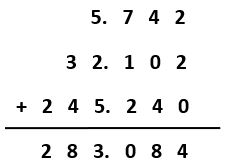## Subtraction of Decimal Numbers

Write the smaller decimal number below the larger decimal number in such manner that the decimal point of the smaller number lies directly below the decimal point of the larger number. Remember that any number of extra zeros can be written at the end of the decimal number. Let's see some examples.

Example 1. Subtract 29.43 from 45.65.

Solution. Arrange both the decimal numbers in tabular format as shown below.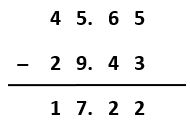Example 2. Subtract 56.6 from 27.605.

Solution. Arrange both the decimal numbers in tabular format as shown below.## Multiplication of Decimal Numbers

1. Ignore the decimal points and multiply the numbers.
2. Count the number of decimal places in number and add them.
3. Start from the right of the product and count number of places equal to the total number of places got in Step. 2 and place the decimal point.

Let's see some examples.

Example 1. Multiply 2.6 and 0.12.

Solution. We have to ignore the decimal points and multiply the numbers.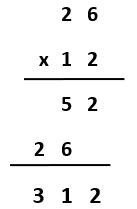Total number of decimal places in both the numbers is 3.

Starting from the right of the product 312 and count up to 3 places, then place the decimal point.

Example 2. Multiply 5.612 and 2.5.

Solution. We have to ignore the decimal points and multiply the numbers.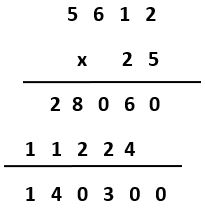Total number of decimal places in both the numbers is 4.

Starting from the right of the product 140300 and count up to 4 places, then place the decimal point.

## Division of Decimal Numbers by Natural Number

Please follow the below mentioned steps to divide decimal number by natural number.

1. Divide the given decimal number by the given natural number in the usual way of division.
2. Put the decimal point in the quotient just after completing the division of the whole number part of the dividend.

Let's see some examples.

Example 1. Divide 4.5 by 3.

Solution.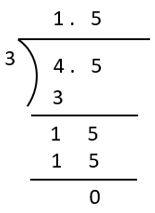So, 4.5 ÷ 3 = 1.5

Example 2. Divide 153.54 by 9.

Solution.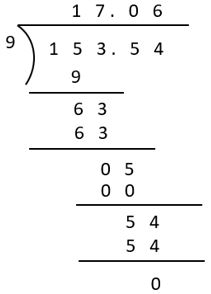153.54 ÷ 9 = 17.06

## Division of Decimal Numbers by Decimal Number

Please follow the below mentioned steps to divide decimal number by decimal number.

1. Convert the divisor decimal number into a natural number by shifting the decimal point by the same number of places in the right direction in the divisor and dividend both.
2. Divide this new dividend by the new divisor in the usual way of division.

Let's see some examples.

Example 1. Divide 145.11 by 0.3.

Solution. 145.11 ÷ 0.3

= 145.110.3 = 1451.13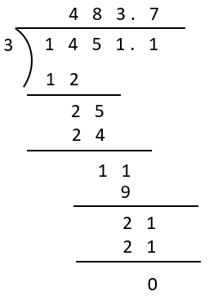So, 145.11 ÷ 0.3 = 483.7

## Decimal Numbers Test

Decimal Numbers Test - 1

Decimal Numbers Test - 2

## Decimal Numbers Worksheet

Decimals Worksheet - 1

Decimals Worksheet - 2

Decimals Worksheet - 3